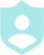###### Example Questions4 + 5 = ?11 - 7 = ?
##### Description :
In this jeopardy style game we will focus on adding, subtracting, dividing, and  multiplying integers. and comparing integers.##### Difficulty :GRID 5 Categories 30 QuestionsDownloadPrintShareAdd
Questions
Number of categoriesQuestions per categoryCategory 1Category 2Category 3
Multiplying IntegersCategory 4
Dividing IntegersCategory 5
Comparing IntegersLayout Preview
 Question Q. 4 + 5 = ? A. 9 Question Q. 15 + 13 = ? A. 28 Question Q. 6 * 3 = ? A. 18 Question Q. 16 ÷ 8 = ? A. 2 Question Q. Describe the relationship between the two integers as < or >. A. 5 > 2 Question Q. 11 - 7 = ? A. 4 Question Q. 27 - 14 = ? A. 13 Question Q. -1 * 7 = ? A. -7 Question Q. 5 ÷ (-5) = ? A. -1 Question Q. scribe the relationship between the two integers as < or >. A. 9 > -6 Question Q. -2 + 5 = ? A. 3 Question Q. -32 + 44 = ? A. 12 Question Q. 6 * (-9) = ? A. -54 Question Q. (-45) ÷ 3 = ? A. -15 Question Q. scribe the relationship between the two integers as < or >. A. -5 < 5 Question Q. 6 - 13 = ? A. -7 Question Q. 41 - 59 = ? A. -18 Question Q. (-8) * (-5) = ? A. 40 Question Q. 77 ÷ 11 = ? A. 7 Question Q. scribe the relationship between the two integers as < or >. A. 1 > 0 Question Q. -4 - 8 =? A. -12 Question Q. -72 + 35 = ? A. -37 Question Q. (-12) * (12 ) = ? A. -144 Question Q. (-48) ÷ (-12) = ? A. 4 Question Q. scribe the relationship between the two integers as < or >. A. -1 < 0 Question Q. -7 - (-9) A. 2 Question Q. -22 - (-53) A. 31 Question Q. 3 * (-5) * (-4) = ? A. 60 Question Q. 65 ÷ (-13) = ? A. -5 Question Q. scribe the relationship between the two integers as < or >. A. -12 > -13
Colors :
Font color
Button color
Background color
Logo:Background Image:Theme Song: